Courses

Test: Linear Algebra

20 Questions MCQ Test RRB JE for Electrical Engineering | Test: Linear Algebra

Description
Attempt Test: Linear Algebra | 20 questions in 60 minutes | Mock test for GATE preparation | Free important questions MCQ to study RRB JE for Electrical Engineering for GATE Exam | Download free PDF with solutions
QUESTION: 1

If A is a non–singular matrix and the eigen values of A are 2 , 3 , -3 then the eigen values of A-1 are:

Solution:
• If λ1 ,λ2 ,λ3 ....λare the eigen values of a non–singular matrix A, then A-1 has the eigen values  1/λ1 ,1/λ2 ,1/λ3 ....1/λn
• Thus eigen values of A-1are 1/2, 1/3, -1/3
QUESTION: 2

If -1, 2, 3 are the eigen values of a square matrix A then the eigen values of A2 are:

Solution:
• If λ1 ,λ2 ,λ3 ....λare the eigen values of  a matrix A, then A2 has the eigen values  λ12 ,λ22 ,λ32 ....λn2
• So, eigen values of Aare 1, 4, 9.
QUESTION: 3

The sum of the eigenvalues of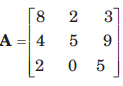is equal to:

Solution:
• Since the sum of the eigenvalues of an n–square matrix is equal to the trace of the matrix (i.e. sum of the diagonal elements)
• So, required sum = 8 + 5 + 5  = 18
QUESTION: 4

If 2, - 4 are the eigen values of a non–singular matrix A and |A| = 4, then the eigen values of adjA are:

Solution: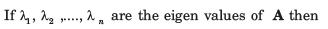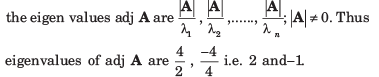QUESTION: 5

If 2 and 4 are the eigen values of A then the eigenvalues of AT are

Solution:

Since, the eigenvalues of A and Aare square so the eigenvalues of AT are 2 and 4.

QUESTION: 6

If 1 and 3 are the eigenvalues of a square matrix A then A3 is equal to:

Solution:
• Since 1 and 3 are the eigenvalues of A so the characteristic equation of A is: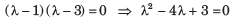• Also, by Cayley–Hamilton theorem, every square matrix satisfies its own characteristic equation. So: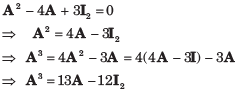QUESTION: 7

If A is a square matrix of order 3 and |A| = 2 then A (adj A) is equal to:

Solution: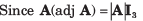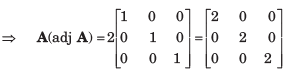QUESTION: 8

If 1, 2 and 5 are the eigen values of the matrix A then |A| is equal to:

Solution:

Since the product of the eigenvalues is equal to the determinant of the matrix so: |A| = 1 x 2 x 5 = 10

QUESTION: 9

If the product of matrices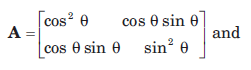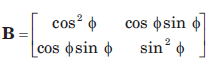is a null matrix, then θ and Ø differ by:

Solution: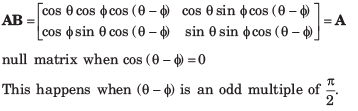QUESTION: 10

If A and B are two matrices such that A +  B and AB are both defined, then A and B are:

Solution:
• Since A + B is defined, A and B are matrices of the same type, say m x n. Also, AB is defined.
• So, the number of columns in A must be equal to the number of rows in B i.e. n = m.
• Hence, A and B are square matrices of the same order.
QUESTION: 11

If A is a 3-rowed square matrix such that |A| = 2, then |adj(adj A2)| is equal to:

Solution: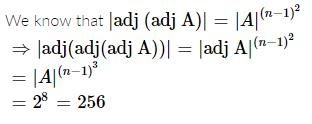QUESTION: 12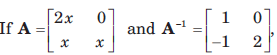then the value of x is:

Solution: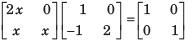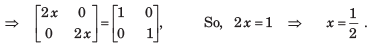QUESTION: 13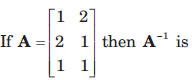Solution:

Inverse matrix is defined for square matrix only.

QUESTION: 14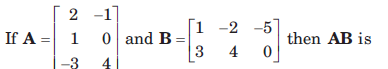Solution: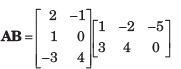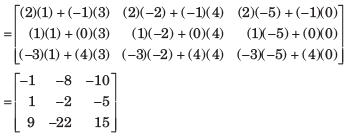QUESTION: 15

A set of linear equations is represented by the matrix equation Ax = b. The necessary condition for the existence of a solution for this system is:

Solution:

A must be invertible.

QUESTION: 16

Select a suitable figure from the four alternatives that would complete the figure matrix.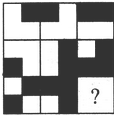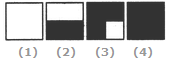Solution:

In each row (as well as each column), the third figure is a combination of all the elements of the first and the second figures.

QUESTION: 17

For a skew symmetric even ordered matrix A of integers, which of the following will not hold true:

Solution:

Determinant of a skew-symmetric even ordered matrix A should be a perfect square.

QUESTION: 18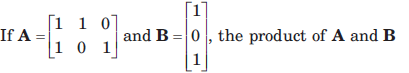Solution: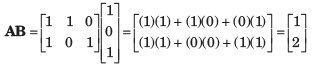QUESTION: 19

Matrix D is an orthogonal matrix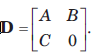The value of B is:

Solution:

For orthogonal matrix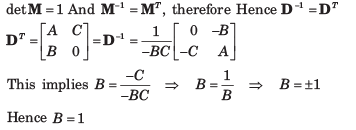QUESTION: 20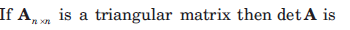Solution:

From linear algebra for Anxn triangular matrix . DetA =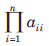The product of the diagonal entries of A.Use Code STAYHOME200 and get INR 200 additional OFF Use Coupon Code

Track your progress, build streaks, highlight & save important lessons and more!

Similar ContentRelated tests